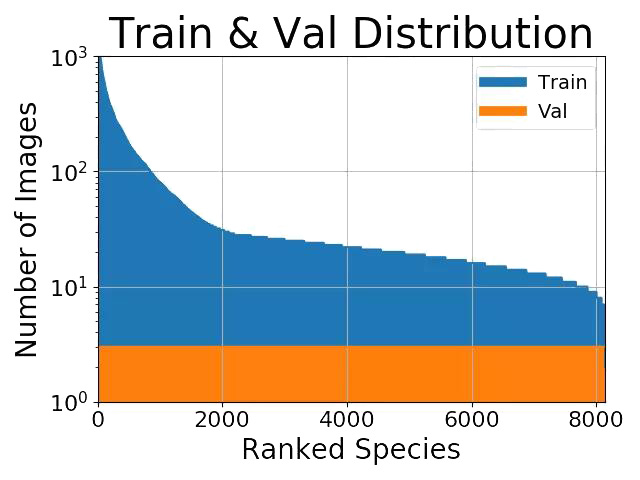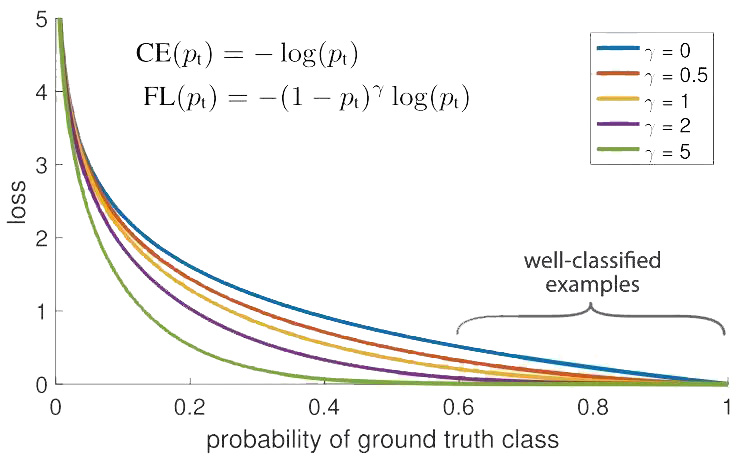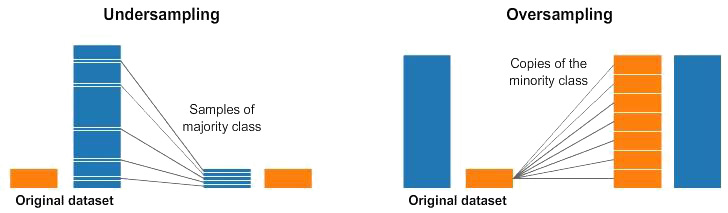# 在深度学习中处理不均衡数据集```import keras
class_weight = {"buy": 0.75,
model.fit(X_train, Y_train, epochs=10, batch_size=32, class_weight=class_weight)``````import keras
from keras import backend as K
import tensorflow as tf

# Define our custom loss function
def focal_loss(y_true, y_pred):
gamma = 2.0, alpha = 0.25
pt_1 = tf.where(tf.equal(y_true, 1), y_pred, tf.ones_like(y_pred))
pt_0 = tf.where(tf.equal(y_true, 0), y_pred, tf.zeros_like(y_pred))
return -K.sum(alpha * K.pow(1. - pt_1, gamma) * K.log(pt_1))-K.sum((1-alpha) * K.pow( pt_0, gamma) * K.log(1. - pt_0))

# Compile our model--电子创新网--